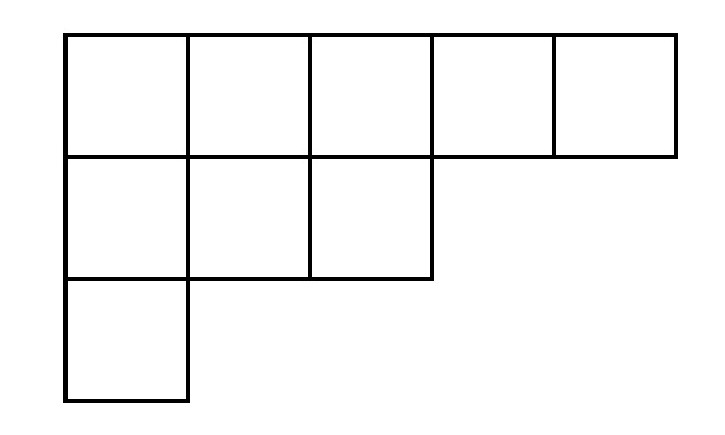# Partitions and the Bloch-Okounkov Theorem

Published:

In this post I will briefly explain what is a partition of a positive integer and how it is possible to relate this theory to quasimodular forms via the Bloch-Okounkov theorem.

## Partitions

In mathematics, a partition of a number $n$ is simply a finite sequence of integers $\lambda_1, \lambda_2, \ldots, \lambda_m$ such that $\lambda_i \geq \lambda_{i+1}$ and

$n = \lambda_1 + \lambda_2 + \cdots + \lambda_m,\quad m\geq 1.$

Equivalently, a partition is a sequence of integers $\lambda = (\lambda_1, \lambda_2, \ldots, \lambda_i, \ldots)$ such that $\lambda_i \geq \lambda_{i+1}$ and $\lambda_i = 0$ for all but finitely many indices $i$. We denote by $\vert \lambda \vert$ the sum of its entries: $\vert \lambda \vert = \lambda_1 + \cdots + \lambda_m$.

For example, $(5,3,2)$ is a partition of the number $10$ and $(3,1,1)$ is a partition of the number $5$.

Exercise. Find all the seven partitions of the number $5$.

We will denotes by $\mathcal{P}$ the set of all partitions. For our purpose, we will be working with function $f : \mathcal{P} \rightarrow \mathbb{Q}$. An example of such function is $f(\lambda) = \vert \lambda \vert$. Given a function $f$ defined over $\mathcal{P}$, it is possible to construct a $q$-power series via the $q$-bracket:

$\langle f \rangle_q := \frac{\sum_{\lambda \in \mathcal{P}} f(\lambda) q^{\vert \lambda \vert}}{\sum_{\lambda \in \mathcal{P}}q^{\vert \lambda \vert}} \in \mathbb{Q}[[q]].$

The theorem of Bloch and Okounkov asserts that this $q$-bracket correspond to the $q$-expansion of a quasimodular form for a certain class of functions $f:\mathcal{P} \rightarrow \mathbb{Q}$ (called shifted symmetric polynomials). To defines these shifted symmetric polynomials, we will need the notion of Young diagram and the Frobenius coordinates of a partition.

## Young Diagrams and Frobenius Coordinates

One way of representing a partition visually is by the use of Young diagrams. The Young diagram of a partition $\lambda = (\lambda_1, \ldots, \lambda_m)$ is given by a series of squares:In the example above, the top row of squares correspond to $\lambda_1 = 5$, the second row correspond $\lambda_2 = 3$ and so on.

Exercise. Draw the Young diagram of $(5,4,3,3,2,1)$.

Now, using the Young diagram of a partition, it is now possible to define the Frobenius coordinates of a partition. Let $\lambda$ be a partition. The Frobenius coordinates of $\lambda$ is the numbers

$(r; a_1, a_2, \ldots, a_r; b_1, b_2, \ldots, b_r)$

such that

• $r$ is the length of the longest principal diagonal in the Young diagram of $\lambda$;
• $a_1 > \ldots > a_i >\ldots > a_r \geq 0$ are the number of cells to the right of the $i$-th cell of this diagonal;
• $b_1 > \ldots > b_i> \ldots > b_r \geq 0$ are the number of cells below the $i$-th cell of this diagonal.

There is nothing better than an example to illustrate this definition. Consider the partition $\lambda = (5, 3, 1)$ with Young diagram:By drawing the longest principal diagonal and counting the number of cells we get:Thus, the Frobenius coordinates of $\lambda = (5, 3, 1)$ is

$(2; 4, 1; 2; 0)$

Exercise. Find the Frobenius coordinates of $\lambda = (5,5,4,1)$.

## Shifted Symmetric Polynomials

In this section, I will give a brief overview of the shifted symmetric polynomials. First, we need to define two invariants of a partition: $P_k(\lambda)$ and $Q_k(\lambda)$ for an integer $k\geq 0$.

Definition. Let $\lambda$ be a partition with Frobenius coordinates $(r; a_1, \ldots, a_r; b_1,\ldots, b_r)$ we define

$P_k(\lambda) := \sum_{i = 1}^r \left[ (a_i + 1/2)^k - (-b_i - 1/2)^k \right], \quad k\geq 0;$ $Q_k(\lambda) := \frac{P_{k-1}(\lambda)}{(k-1)!} + \beta_k,\quad k\geq 1;$

where $\beta_k$ is the $k$-th coefficients of the expansion of $\frac{z/2}{\mathrm{sinh(z/2)}}$ around $z=0$.

Definition. A shifted symmetric polynomial is a function $f:\mathcal{P} \rightarrow \mathbb{Q}$ living in $\mathcal{R} := \mathbb{Q}[Q_1, Q_2, \ldots, Q_k, \ldots]$. We define $\mathcal{R}_k \subset \mathcal{R}$ to be the weight $k$ graded component of $\mathcal{R}$, that is the subring generated by the weight $k$ homogenenous monomials:

$\mathcal{R}_k = \langle Q_{k_1}^{m_1}\cdots Q_{k_N}^{m_N} : k_i, m_i\geq 1, k_1 m_1 + \cdots + k_N m_N = k \rangle$

Theorem (Bloch-Okounkov). For any $f\in \mathcal{R}_k$, the $q$-bracket $\langle f \rangle_q$ correspond to the $q$-expansion of a quasimodular forms of weight $k$ for $\mathrm{SL}_2(\mathbb{Z})$.

This theorem is interesting as it relates two apparently different concept: partitions and (quasi)modular forms. In particular, the Bloch-Okounkov theorem can be seen as a tool for studying partitions via quasimodular forms and vice versa.

A proof of this theorem can be found in the the main reference for this post:

Don Zagier, Partitions, quasimodular forms and the Bloch-Okounkov theorem

In the next weeks, I will be working on the SageMath implementation of the objects described above. In a later version of Sage, it should be possible to do computations with quasimodular forms and to compute the $q$-bracket of a shifted symmetric polynomial.